# Hectogon

Regular hectogon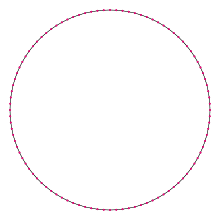A regular hectogon
Type Regular polygon
Edges and vertices 100
Schläfli symbol {100}, t{50}, tt{25}
Coxeter diagram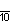Symmetry group Dihedral (D100), order 2×100
Internal angle (degrees) 176.4°
Dual polygon Self
Properties Convex, cyclic, equilateral, isogonal, isotoxal

In geometry, a hectogon or hecatontagon or 100-gon  is a hundred-sided polygon. The sum of any hectogon's interior angles is 17640 degrees.

## Regular hectogon

A regular hectogon is represented by Schläfli symbol {100} and can be constructed as a truncated pentacontagon, t{50}, or a twice-truncated icosipentagon, tt{25}.

One interior angle in a regular hectogon is 17625°, meaning that one exterior angle would be 335°.

The area of a regular hectogon is (with t = edge length)The circumradius of a regular hectogon isBecause 100 = 22 × 52, the number of sides is neither a product of distinct Fermat primes nor a power of two. Thus the regular hectogon is not a constructible polygon. Indeed, it is not even constructible with the use of neusis or an angle trisector, as the number of sides is neither a product of distinct Pierpont primes, nor a product of powers of two and three.

## Symmetry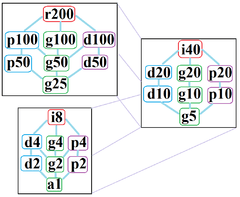The symmetries of a regular hectogon. Light blue lines show subgroups of index 2. The 3 boxed subgraphs are positionally related by index 5 subgroups.

The regular hectogon has Dih100 dihedral symmetry, order 200, represented by 100 lines of reflection. Dih100 has 8 dihedral subgroups: (Dih50, Dih25), (Dih20, Dih10, Dih5), (Dih4, Dih2, and Dih1). It also has 9 more cyclic symmetries as subgroups: (Z100, Z50, Z25), (Z20, Z10, Z5), and (Z4, Z2, Z1), with Zn representing π/n radian rotational symmetry.

John Conway labels these lower symmetries with a letter and order of the symmetry follows the letter. r200 represents full symmetry and a1 labels no symmetry. He gives d (diagonal) with mirror lines through vertices, p with mirror lines through edges (perpendicular), i with mirror lines through both vertices and edges, and g for rotational symmetry.

These lower symmetries allows degrees of freedom in defining irregular hectogons. Only the g100 subgroup has no degrees of freedom but can seen as directed edges.

## Hectogram

A hectogram is an 100-sided star polygon. There are 19 regular forms given by Schläfli symbols {100/3}, {100/7}, {100/9}, {100/11}, {100/13}, {100/17}, {100/19}, {100/21}, {100/23}, {100/27}, {100/29}, {100/31}, {100/33}, {100/37}, {100/39}, {100/41}, {100/43}, {100/47}, and {100/49}, as well as 30 regular star figures with the same vertex configuration.

Picture Interior angle Picture Interior angle Picture Interior angle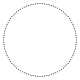{100/3}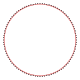{100/7}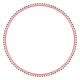{100/11}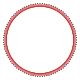{100/13}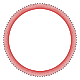{100/17}{100/19} 169.2° 154.8° 140.4° 133.2° 118.8° 111.6°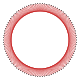{100/21}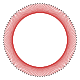{100/23}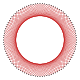{100/27}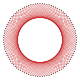{100/29}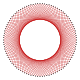{100/31}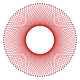{100/37} 104.4° 97.2° 82.8° 75.6° 68.4° 46.8°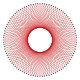{100/39}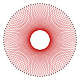{100/41}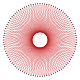{100/43}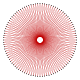{100/47}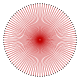{100/49} 39.6° 32.4° 25.2° 10.8° 3.6°

## References

1. Gorini, Catherine A. (2009), The Facts on File Geometry Handbook, Infobase Publishing, p. 110, ISBN 9781438109572.
2. Constructible Polygon
3. http://www.math.iastate.edu/thesisarchive/MSM/EekhoffMSMSS07.pdf
4. The Symmetries of Things, Chapter 20
5. 19 = 50 cases - 1 (convex) - 10 (multiples of 5) - 25 (multiples of 2)+ 5 (multiples of 2 and 5)